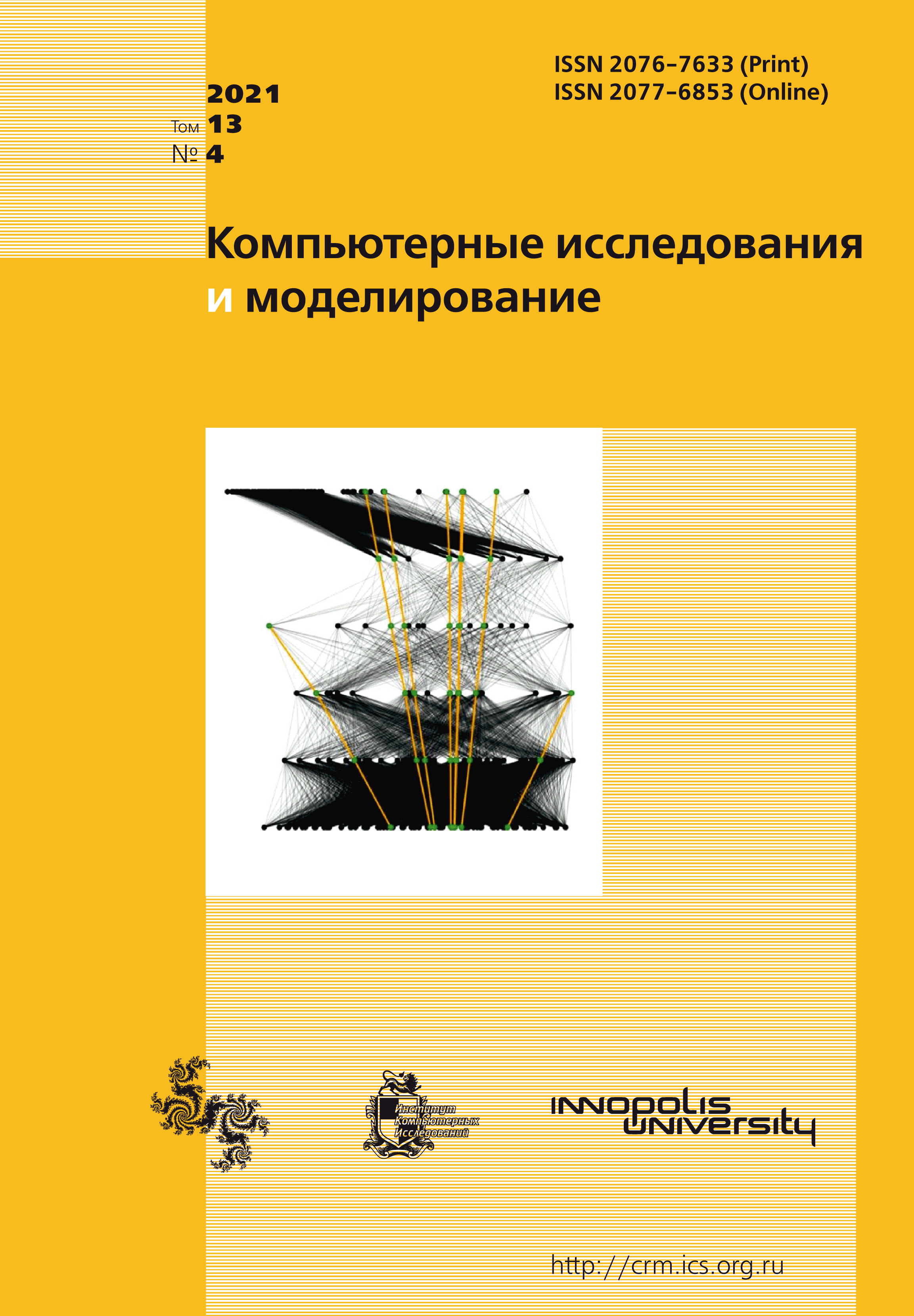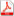Latest issue Issue 4, 2021 Vol. 13

# All issues

Estimation of the probability of spontaneous synthesis of computational structures in relation to the implementation of parallel information processingpdf (592K)

We consider a model of spontaneous formation of a computational structure in the human brain for solving a given class of tasks in the process of performing a series of similar tasks. The model is based on a special definition of a numerical measure of the complexity of the solution algorithm. This measure has an informational property: the complexity of a computational structure consisting of two independent structures is equal to the sum of the complexities of these structures. Then the probability of spontaneous occurrence of the structure depends exponentially on the complexity of the structure. The exponential coefficient requires experimental determination for each type of problem. It may depend on the form of presentation of the source data and the procedure for issuing the result. This estimation method was applied to the results of a series of experiments that determined the strategy for solving a series of similar problems with a growing number of initial data. These experiments were described in previously published papers. Two main strategies were considered: sequential execution of the computational algorithm, or the use of parallel computing in those tasks where it is effective. These strategies differ in how calculations are performed. Using an estimate of the complexity of schemes, you can use the empirical probability of one of the strategies to calculate the probability of the other. The calculations performed showed a good match between the calculated and empirical probabilities. This confirms the hypothesis about the spontaneous formation of structures that solve the problem during the initial training of a person. The paper contains a brief description of experiments, detailed computational schemes and a strict definition of the complexity measure of computational structures and the conclusion of the dependence of the probability of structure formation on its complexity.

Keywords: algorithm, computational structure, iterative structure, complexity, probability, engineering psychology, statistics
Citation in English: Koganov A.V. Estimation of the probability of spontaneous synthesis of computational structures in relation to the implementation of parallel information processing // Computer Research and Modeling, 2021, vol. 13, no. 4, pp. 677-696
Citation in English: Koganov A.V. Estimation of the probability of spontaneous synthesis of computational structures in relation to the implementation of parallel information processing // Computer Research and Modeling, 2021, vol. 13, no. 4, pp. 677-696
DOI: 10.20537/2076-7633-2021-13-4-677-696

Full-text version of the journal is also available on the web site of the scientific electronic library eLIBRARY.RU

The journal is included in the Russian Science Citation Index

The journal is included in the List of Russian peer-reviewed journals publishing the main research results of PhD and doctoral dissertations.

International Interdisciplinary Conference "Mathematics. Computing. Education"

The journal is included in the RSCI

Indexed in Scopus